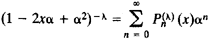Ultraspherical Polynomial

Ultraspherical Polynomial

(or Gegenbauer polynomial). Ultraspherical polynomials are a special family of polynomials of successively increasing degree. For n = 0,1, 2,. . ., the ultraspherical polynomialsof degree n are the coefficients of an in the power series expansion of the functionUltraspherical polynomials are orthogonal on the interval [–1, + 1] with respect to the weight function (1 – x2)λ–(½). Ultra spherical polynomials constitute a special case of Jacobi polynomials.

References in periodicals archive ?
The special case of [beta] = [alpha] of the Jacobi polynomial is called Ultraspherical polynomial and is denoted by [P.
n](x) of the first and second kinds respectievely are special Ultraspherical polynomials.
Balazs  investigated the above problem on the interval [-1,1], when the nodes are the zeros of the ultraspherical polynomial [P.
BALAZS, Weighted (0, 2) -interpolation on the zeros of the ultraspherical polynomials, (in Hungarian: Sulyozott (0, 2)-interpolacio ultraszferikus polinom gyokein), MTA IILoszt.
In  Olver and Townsend presented a fast spectral method for solving linear differential equations using bases of ultraspherical polynomials, which has subsequently been exploited in Chebfun  and ApproxFun .
Complex and distributional weights for sieved ultraspherical polynomials.
LORCH, Inequalities for ultraspherical polynomials and the gamma function, J.
ISMAIL, A generalization of ultraspherical polynomials, in Studies in Pure Mathematics, P.
14] --, Zeros of ultraspherical polynomials and the Hilbert-Klein formulas, J.
uncertainty principle, self-adjoint operators, symmetric operators, normal operators, periodic functions, ultraspherical polynomials, sphere.
Ultraspherical polynomials are solutions of the differential equations [L.

Site: Follow: Share:
Open / Close